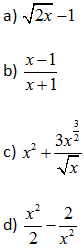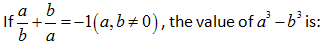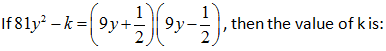# MCQ Questions for Class 9 Maths Chapter 2 Polynomials with Answers

## MCQ Questions for Class 9 Maths Chapter 2 Polynomials with Answers

MCQs from Class 9 Maths Chapter 2 – Polynomials are provided here to help students prepare for their upcoming Maths exam.

MCQs from CBSE Class 9 Maths Chapter 2: Polynomials

1) x2-2x+1 is a polynomial in:
a. One Variable
b. Two Variables
c. Three variable
d. None of the above

Explanation: x2-2x+1 can be written as x2-2x1+1x0. Hence, we can see that x is the only variable having powers as whole numbers: 2,1 and 0.

2) The coefficient of x2 in 3x3+2x2-x+1 is:
a. 1
b. 2
c. 3
d. -1

Explanation: The coefficient of x2 in equation 3x3+2x2-x+1 is the multiple of x2.

3) A binomial of degree 20 in the following is:
a. 20x + 1
b. x/20 + 1
c. x20 +1
d. x2+20

Explanation: A polynomial having two terms and the highest degree 20 is called a binomial of degree 20.

4) The degree of 4x3-12x2+3x+9 is
a. 0
b. 1
c. 2
d. 3

Explanation: The degree is the highest power of a variable in an equation.

5) x2 – x is ________ polynomial.
a. Linear
c. Cubic
d. None of the above

Explanation: A polynomial of degree two is known as quadratic polynomial.

6) x – x3 is a ________ polynomial.
a. Linear
c. Cubic
d. None of the above

Explanation: A polynomial of degree three is known as cubic polynomial.

7) 1+3x is a _________ polynomial.
a. Linear
c. Cubic
d. None of the above

Explanation: A polynomial of degree one is known as linear polynomial.

8) The value of f(x) = 5x−4x2+3 when x = -1, is:
a. 3
b. -12
c. -6
d. 6

Explanation: When x= -1

f(x)=5x−4x2+3

f(−1)=5(−1) −4(−1)2+3

=−5–4+3

=−6

9) The value of p(t) = 2+t+2t2−t3 when t=0 is
a. 2
b. 1
c. 4
d. 0

Explanation: p(0)=2+0+2(0)2–(0)3=2

10) The zero of the polynomial f(x) = 2x+7 is
a. 2/7
b. -2/7
c. 7/2
d. -7/2

Explanation: f(x)=2x+7

⇒2x+7=0

⇒2x=−7

⇒x=−7/2

∴x = −7/2 is a zero polynomial of the polynomial f(x).

11) A polynomial with one degree is called:
a) Linear polynomial
c) Monomial
d) Binomial

(b)

12) Which one of the following is a polynomial?(c)

13) √3 is a polynomial of degree:
a) 2
b) 0
c) 1
d) 1/2

(b)

14) Degree of the polynomial 7x5 + 8x2 – 5x + 3 is:
a) 1
b) 3
c) 2
d) 5

(d)

15) What is the degree of a zero polynomial?
a) 0
b) 1
c) Any natural number
d) Not defined

(d)

16) The value of the polynomial 7x4 + 3x2 – 4, when x = – 2 is:
a) 100
b) 110
c) 120
d) 130

(c)

17) The zero of the polynomial p(x) = -9x + 9is:
a) 0
b) -9
c) -1
d) 1

(d)

18) √12 X √15 is equal to:
a) 5√6
b) 6√5
c) 10√5
d) √25

(b)

19) If y97 + 97 is divided by y + 1, the remainder is:
a) 0
b) 1
c) 95
d) 96

(d)

20) If x + 1 is a factor of the polynomial 2x2 + kx, then the value of k is:
a) -3
b) 4
c) 2
d) -2

(c)

21) The value of 992 – 982 is:
a) 1
b) 197
c) 187
d) 207

(b)

22) One of the factors of (1 + 7x)2 + (49x2 – 1) is:
a) x – 7
b) 7 – x
c) 7x – 1
d) 14x

(d)

23) The factorization of 6x2 + 11x + 3 is:
a) (3x + 1) (2x + 3)
b) (x + 1) (2x + 3)
c) (x + 3) (2x + 1)
d) (3x + 3) (x + 1)

(a)

24)a) 1
b) –1
c) 0
d) 1/2

(c)

25)a) 0
b) 1/4
c) 1/2
d) 1/√2